Courses

# Test: Turing Machine & Halting

## 10 Questions MCQ Test Theory of Computation | Test: Turing Machine & Halting

Description
This mock test of Test: Turing Machine & Halting for Computer Science Engineering (CSE) helps you for every Computer Science Engineering (CSE) entrance exam. This contains 10 Multiple Choice Questions for Computer Science Engineering (CSE) Test: Turing Machine & Halting (mcq) to study with solutions a complete question bank. The solved questions answers in this Test: Turing Machine & Halting quiz give you a good mix of easy questions and tough questions. Computer Science Engineering (CSE) students definitely take this Test: Turing Machine & Halting exercise for a better result in the exam. You can find other Test: Turing Machine & Halting extra questions, long questions & short questions for Computer Science Engineering (CSE) on EduRev as well by searching above.
QUESTION: 1

### Which of the following regular expression resembles the given diagram?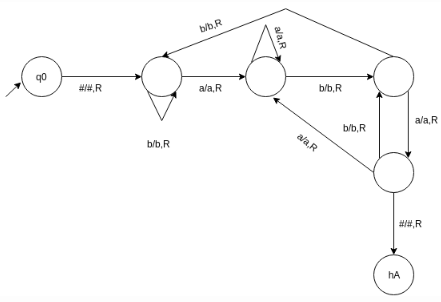Solution:

The given diagram is a transition graph for a turing machine which accepts the language with the regular expression {a,b}*{aba}.

QUESTION: 2

### Construct a turing machine which accepts a string with ‘aba’ as its substring.

Solution:

The language consist of strings with a substring ‘aba’ as fixed at its end and the left part can be anything including epsilon. Thus the turing machine uses five states to express the language excluding the rejection halting state which if allowed can modify the graph as:

QUESTION: 3

### The number of states required to automate the last question i.e. {a,b}*{aba}{a,b}* using finite automata:

Solution:

The finite automata can be represented as: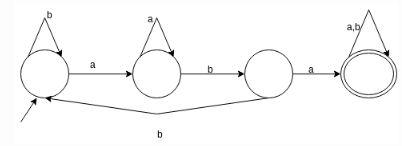QUESTION: 4

The machine accept the string by entering into hA or it can:

Solution:

Three things can occur when a string is tested over a turing machine:
a) enter into accept halting state
b) enter into reject halting state
c) goes into loop forever

QUESTION: 5

d(q,X)=(r,Y,D) where D cannot be: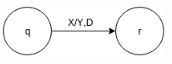Solution:

D represents the direction in which automata moves forward as per the queue which surely cannot be a starting variable.

QUESTION: 6

Which of the following can accept even palindrome over {a,b}

Solution:

A language generating strings which are palindrome is not regular, thus cannot b represented using a finite automaton.

QUESTION: 7

Which of the functions can a turing machine not perform?

Solution:

Different turing machines exist for operations like copying a string, deleting a symbol, inserting a symbol and accepting palindromes.

QUESTION: 8

If T1 and T2 are two turing machines. The composite can be represented using the expression:

Solution:

If T1 and T2 are TMs, with disjoint sets of non halting states and transition function d1 and d2, respectively, we write T1T2 to denote this composite TM.

QUESTION: 9

The following turing machine acts like: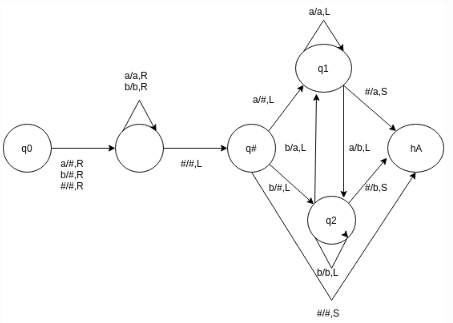Solution:

A turing machine does the deletion by changing the tape contents from yaz to yz, where y belongs to (S U {#})*.

QUESTION: 10

What does the following transition graph shows: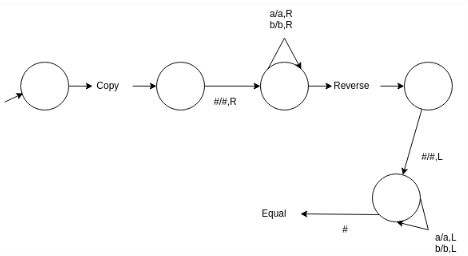Solution:

The composite TM accepts the language of palindromes over {a, b} by comparing the input string to its reverse and accepting if and only if the two are equal.